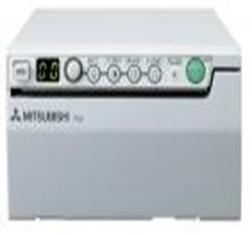Tìm kiếm nâng cao

 Chọn Danh mục=Thiết bị y tế==Khoa ngoại====Máy sốc tim====Máy gây mê, kèm thở====Máy thở====Đèn mổ====Dao mổ điện====Máy Monitor theo dõi bệnh nhân====Bồn rửa tay phẫu thuật tiệt trùng====Máy làm ấm dịch truyền====Bàn mổ==Khoa chẩn đoán hình ảnh====Máy X-Quang kỹ thuật số====Máy rửa phim X-Quang tự động====Máy X-quang cao tần====Máy in siêu âm====Máy siêu âm========Máy siêu âm honda========Máy siêu âm sonosite==Khoa Tai Mũi Họng====HT Nội soi Tai Mũi Họng + Ghế bệnh nhân==Khoa sản====Máy hút dịch====Monitor sản khoa====Doppler tim thai====Máy làm ấm trẻ sơ sinh====Lồng ấp====Đèn điều trị vàng da====Máy soi cổ tử cung==Khoa xét nghiệm====Máy phân tích huyết học====Máy phân tích sinh hóa tự ðộng====Máy điện di====Máy đo độ đông máu====Máy phân tích sinh hóa bán tự ðộng==Khoa thăm dò chức năng====Máy đo sơ vữa động mạch====Máy đo độ bão hòa oxy trong máu====Máy điện não====Máy siêu âm====Máy điện tim====Holter điện tim====Holter huyết áp====Thảm lăn gắng sức====Máy đo chức năng hô hấp========Máy đo chức năng hô hấp==Khoa phục hồi chức năng====Máy điều trị điện từ trường====Máy điều trị bỏng====Buồng Oxy cao áp==Vật tư tiêu hao====Đinh, nẹp, vít xương ….==Thiết bị chung====Máy kích thích từ xuyên sọ====Tủ bảo quản tử thi====Bể tải lát cắt====Máy vùi mô chuyên dụng====Máy xử lý mô====Máy nhuộm==Hệ thống xử lý chất thải====Lò đốt rác=Thiết bị an ninh==Máy phá sóng điện thoại==Máy đọc tài liệu==Máy kiểm tra tài liệu==Camera====Camera quan sát dưới nước====Camera giám sát====Camera theo dõi ngày đêm====Camera đường cao tốc==Súng bắn tốc độ==Xe chuyên dụng====Dành cho tác chiến====Dành cho phòng trống khủng bố====Dành cho cứu hỏa==Thiết bị thu phát âm thanh==Máy nghe xuyên tường==Thiết bị dò kim loại==Thiết bị dò hơi nổ==Thiết bị dò tìm ma tuý==Thiết bị kiểm tra bưu kiện, dò bom thư=Thiết bị giáo dục==Đồ chơi ngoài trời====Thiết bị xích đu====Thiết bị nhà bóng====THIẾT BỊ ĐU QUAY MÂM====THIẾT BỊ BẬP BÊNH THÚ====THIẾT BỊ THANG LEO THỂ DỤC====THÚ NHÚN LÒ XO====CẦU TRƯỢT====BỘ LIÊN HOÀN==ĐỒ CHƠI TRONG LỚP====THIẾT BỊ GIÁO DỤC THỂ CHẤT====CÁC BỘ HỌC TOÁN MẦM NON====ĐỒ CHƠI ÂM NHẠC====BỘ ĐỒ CHƠI ĐỒ DÙNG==THIẾT BỊ NHÀ BẾP==BÀN GHẾ MẦM NON====BÀN GHẾ MẦM NON====GIƯỜNG NGỦ MẦM NON====BÀN GHẾ THƯ VIỆN====BẢNG TIN MẦM NON==KỆ MẦM NON====KỆ DÉP, ĐỰNG ĐỒ====KỆ GÓC THƯ GIÃN==THIẾT BỊ TIỂU HỌC====BẢNG NHÓM====TỦ ĐỰNG THIẾT BỊ====BẢNG PHÔ====TI VI====ĐẦU ĐỌC ĐĨA====RADIO-CASTSETE====QUẢ ĐỊA CẦU====NAM CHÂM====NẸP TREO TRANH====GIÁ TREO TRANH====MÁY VI TÍNH====MÁY CHIẾU====BỘ MẪU TƠ SỢI====BỘ DỤNG CỤ VẬT LIỆU CẮT THÊU LỚP 4====BỘ THỰC HÀNH TOÁN VÀ TIẾNG VIỆT LỚP 1====BỘ LẮP MẠCH ĐIỆN ĐƠN GIẢN====BỘ LẮP GHÉP MÔ HÌNH KĨ THUẬT LỚP 4====BỘ THỰC HÀNH TOÁN 2====BỘ LẮP GHÉP MÔ HÌNH KỸ THUẬT LỚP 5====BỘ THỰC HÀNH TOÁN LỚP 5====BẢNG PHỤ BẰNG NHỰA====BỘ THỰC HÀNH TOÁN LỚP 4====THƯỚC NHÔM 1M====BÚT THÔNG MINH TEDDY====TRANH TIẾNG VIỆT LỚP 2====TRANH TIẾNG VIỆT LỚP 4====TRANH TỰ NHIÊN XÃ HỘI LỚP 1====TRANH THỦ CÔNG LỚP 3====HỘP DỤNG CỤ MÀU VẼ====MÔ HÌNH BÁNH XE NƯỚC====TRANH TỰ NHIÊN XÃ HỘI LỚP 2====BỘ THÍ NGHIỆM SỰ DÃN NỞ VÌ NHIỆT CỦA CHẤT LỎNG====TRANH LỊCH SỬ VÀ ĐỊA LÝ LỚP 4====TRANH TẬP ĐỌC LỚP 1====BÚT THÔNG MINH K- 500==THIẾT BỊ TRUNG HỌC CƠ SỞ====BẢNG NHÓM====TỦ ĐỰNG THIẾT BỊ==THIẾT BỊ TRUNG HỌC PHỔ THÔNG=Thiết bị khoa học==Thiết bị phòng thí nghiệm====Pipettes====Máy cất nước====Tủ ấm CO2====Tủ cấy vi sinh====Tủ sấy====Tủ bảo quản huyết thanh và tủ lạnh âm sâu====Tủ lạnh bảo quản máu, vaccine====Máy làm tan huyết thanh====Tủ bảo quản và lắc túi tiểu cầu====Tủ hốt xét nghiệm====Máy đo Ph,COD,DO====Quang phổ đo chỉ tiêu nước====Testkit đo chỉ tiêu nước====Dụng cụ lấy mẫu==Xe thu mẫu tại hiện trường==Life Sciences====Thiết bị====Hoá chất và Kít====Đồ tiêu hao==BioProcess====Lọc tiếp tuyến====Lọc cột====Kiểm soát môi trường sản xuất==Thiết bị chung====Lọc nước siêu sạch====Trộn mẫu========Khuấy từ không gia nhiệt========Khuấy từ gia nhiệt========Khuấy cơ========Khuấy điện========Lắc ngang========Lắc ổn nhiệt========Lắc tròn====Nghiền========Nghiền khô========Đồng hoá mẫu====Ổn nhiệt========Bể ổn nhiệt========Bếp gia nhiệt====Cất quay====Tủ ấm - sấy==Thiết bị phân tích====Phản ứng====Điện hoá====Đo nhiệt lượng Chọn hãng ACOMAAtmosBOW TECHCamiCEABISCONTROL XDAESUNGGENEXGIMAHOSPITEXINNOVATIVIEWKANMEDKEISEILABTECHLuvivaMAGSTIMMEDGYNMEDICONETMedifaMitsubishiREGULARexmedRIMSAStephanToshibaTRISMED

# Thống kê

 Truy cập: 960682 Trực tuyến: 4Máy in siêu âm nhiệt đơn sắc P-95W Mã sản phẩm:   Nhà sản xuất:   Xuất xứ:   Bảo hành: Chia sẻ qua:Mô tả sản phẩm

## Monochrome Printer P95DWHigh Quality, Quick & Compact

A high quality, quick and compact digital monochrome printer with worldwide compatibility for medical applications. User-friendly controls with simple, analog-like operation are conveniently located. The compact and lightweight Mitsubishi P-95DW incorporates internal 16-bit ASIC image processing which simultaneously achieves superior image quality with faster speed. A high density 325 dpi thermal head and resolution of 1280 x 1280 pixels results in exceptionally sharp reproduction of even the most intricate details.

### Features

·  High speed printing

·  High-density 325 dpi thermal head

·  Enhanced user-friendly controls conveniently located on front panel

·  Streamlined design with small footprint

·  Jam-free reliability

·  USB 2.0 interface

·  Worldwide compatibility

Sản phẩm liên quan

Đánh giá sản phẩm qua facebookCÔNG TY DỰ ÁN HỖ TRỢ LIÊN HỆTin tứcTin khuyến mãiSự kiệnMiền bắcMiền trungMiền namHình thức thanh toánThông tin liên hệ

Design by: ATG

Hotline: 0904.987.576

Hỗ trợ bán hàng trực tuyến

 Ms Nhung 0902987576Mr.Hà 0904987576CSKH 046 654 2704